# Introduction to Wavelets and Filter Banks

Although the history of filter banks and wavelets has been brief, they have had a large impact on signal and image processing. Filter banks, which came first, are used for a number of different audio (1D), images (2D), and video (2D frames changed 30 times every second for interlaced video) applications.

Filtering is a linear time-invariant process. For a process to be linear, the change to the input vs. the change to the output must be linear or the same (example: if y(n) = x(n) then 2y(n) = 2x(n) or 3y(n) = 3x(n)). For a process to be time-invariant, any change related to the time of the input results in an equivalent change in the time of the output (example if y(t) = x(t) then y(t-2) = x(t-2)).

Filtering is a process performed on an input vector or signal x ([…, x(-1), x(0), x(1),…]). It results in an output vector y ([…, y(-1), y(0), y(1),…]), which is the convolution of x with filter vector h ([h(0), h(1),…]) in the discrete time-domain. Mathematically, this may be expressed by the equationor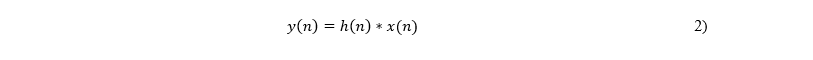where n is discrete time and the “*” is the called the convolution operator. Discrete time n = t/T, where t is continuous time and T is the sampling period. Equation 1 and 2 can be represented as a block diagram as shown in Figure 1 where the h(n), also known as the impulse response, is used as an indicator that the figure represents filtering in the discrete time-domain.In order to look at the filtering process in the frequency-domain, we must perform the Fourier transformation of the vectors, first. Mathematically, that is done by doing the following: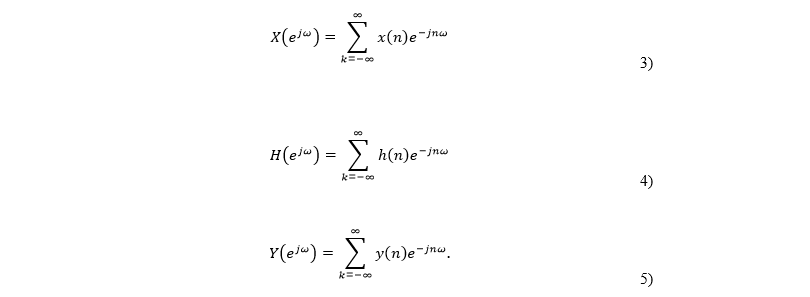This yields the equation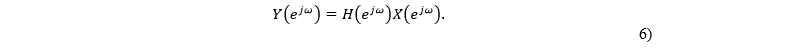That is the multiplication of the H, the frequency response, and X is equal to the Y. In reduced notation, Y (ω) = H(ω) X(ω) and may be illustrated in block diagram form as shown in Figure 2.When the frequency response is expressed as the output vs. the input in the z domain where z = ejω (or H(ω) = Y(ω) / X(ω)), it is referred to as the transfer function.

Two common filters used for processing signal vectors are lowpass filters (or summing filters labeled h0) and highpass filters (or difference filters labeled h1). Lowpass filters allow low frequency signals to pass through while attenuating high frequency signals. The highpass filter does the exact opposite. The simplest lowpass and highpass discrete filters are the Haar filters, developed by Alfred Haar. The lowpass filter is a two sample (or tap) filter vector h0 = [1, 1] while one form of the highpass filter is h1 = [1, -1].

Using the vector in Figure 3 as the input signal to both a lowpass and highpass Haar filter, the results in Figure 4a and 4b are obtained.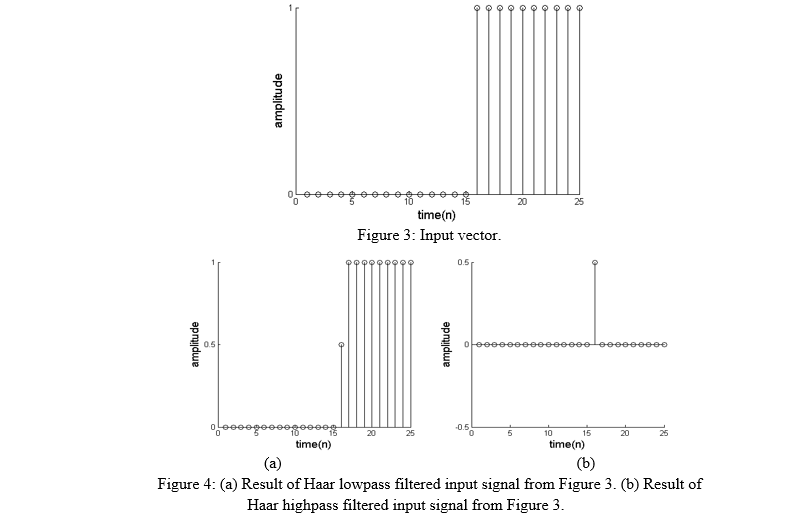It is important to note that there is only one area that contains high frequency data in Figure 3, and it occurs between time samples 15 and 16. It is the quick transition from 0 to 1. Low frequency data has no change from one sample to the succeeding sample. This means all other areas in Figure 3 contain low frequencies. Given the characteristics of Haar filters and the input vector, it is possible to explain the results seen in Figure 4. There is some attenuation in Figure 4a only at time sample 16 because the low pass filter eliminated some of the high frequency data. As show in Figure 4b, the high frequency filter encountered only one occurrence of high frequency data at time sample 16.

Filter banks are a set of filters used in conjunction to perform a single signal processing task. They are typically chosen to separate the frequency spectrum into small subbands for a number of applications such as analysis, compression, data hiding, and image enhancing. By that definition, using Haar filters to separate the spectrum of frequencies in Figure 3 into two subbands, a lowpass subband and a highpass subband, makes them collectively a filter bank.

Wavelets are really filter banks in the continuous domain. This means Equation 1 becomes Equation 7 in a continuous domain.orTo those working in the continuous domain, a process with characteristics similar to a lowpass filter is called a scaling function and is labeled as ϕ(t), while a process with characteristics similar to a highpass filter is called a wavelet function and is labeled as ω(t). These functions were called wavelets because they resembled waves. Figure 5 shows an example of what those working in this area saw that made them call the function a wavelet.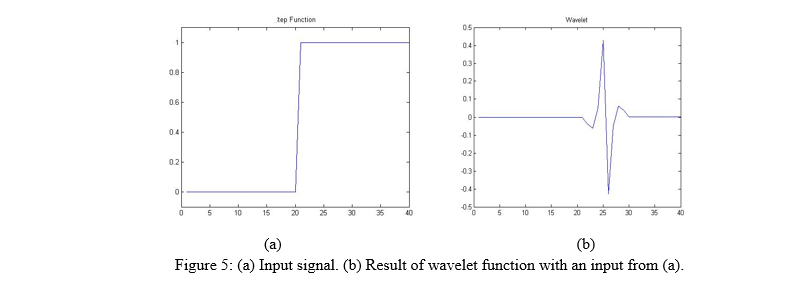Because I will there has been such a large shift towards digital technology the remainder of this paper will only address this topic in the discrete time-domain rather than in the continuous or analog domain.